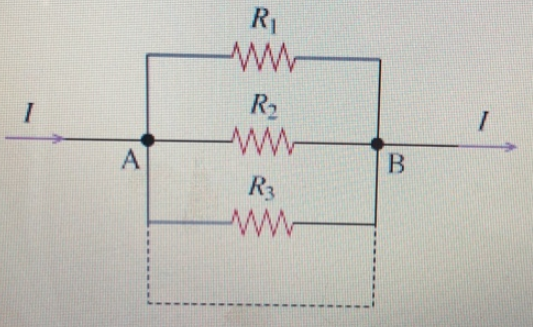# Problem: For the set-up shown, find the equivalent resistance between points A and B.

###### FREE Expert Solution

Equivalent resistance for parallel resistors:

$\overline{)\frac{\mathbf{1}}{{\mathbf{R}}_{\mathbf{eq}}}{\mathbf{=}}\frac{\mathbf{1}}{{\mathbf{R}}_{\mathbf{1}}}{\mathbf{+}}\frac{\mathbf{1}}{{\mathbf{R}}_{\mathbf{2}}}}$

The resistors are in parallel.###### Problem Details

For the set-up shown, find the equivalent resistance between points A and B.